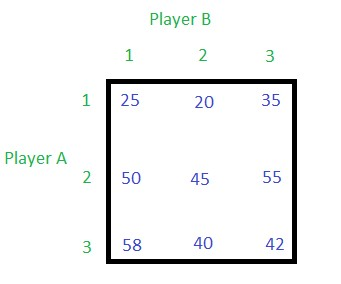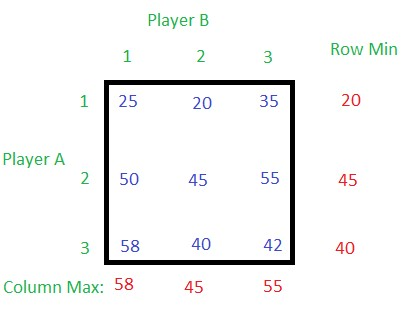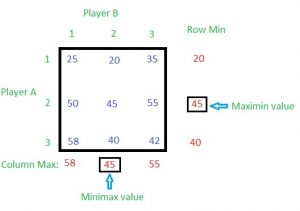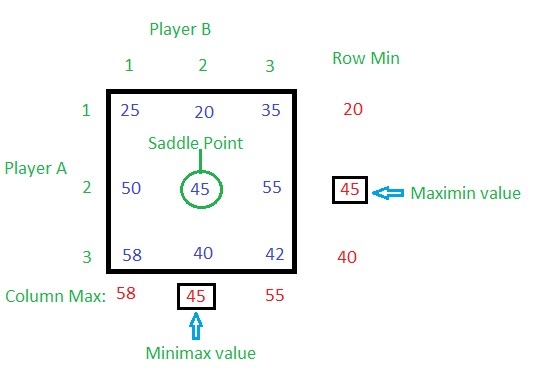GFG App
Open AppBrowser
Continue

# Game Theory (Normal form game) | Set 2 (Game with Pure Strategy)

Game Theory (Normal – form game) | Set 1 (Introduction)

Please go through the above article before proceeding.

Given a payoff matrix. The task is to find the optimum strategies of the players.Solution:
Player A is having 3 strategies – 1, 2 and 3, and player B is also having 3 strategies – 1, 2 and 3.

• Step 1: Find row minimum values for each row and column maximum value for each column.20, 45 and 40 are the minimum values in the first, second and third row respectively. 58, 45 and 55 are the maximum values in first, second and third column respectively.
• Step 2: Find maximum of the row minimum (Row Min) values and minimum of the column maximum (Column Max) values.The maximum of Row Min value is called maximin value and the minimum of Column Max value is called minimax.

Here the maximin value is equal to the minimax value, so this game has a saddle point at the cell corresponding to Row 2 and Column 2 (see the figure below).Therefore, the value of the game (V) is 45. Optimal probabilities of both the players for each strategy,

A [P1, P2, P3] = A [0, 1, 0]
B [Q1, Q2, Q3] = B [0, 1, 0]

where,
P1, P2 and P3 are probabilities of strategy 1, 2 and 3 respectively for player A.
Q1, Q2 and Q3 are probabilities of strategy 1, 2 and 3 respectively for player B
For both the players the total probability is 1.

My Personal Notes arrow_drop_up